Since 12 August, 2008.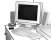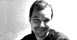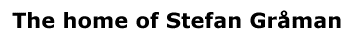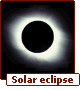# Extra Dimensions

Superstrings live in a 10-dimensional spacetime, but we observe a 4-dimensional spacetime. Somehow we need to link the two if superstrings are to describe our universe. To do this we curl up the extra 6 dimensions into a small compact space. If the size of the compact space is of order the string scale (10-33 cm) we wouldn't be able to detect the presence of these extra dimensions directly - they're just too small. The end result is that we get back to our familiar (3+1)-dimensional world, but there is a tiny "ball" of 6-dimensional space associated with every point in our 4-dimensional universe. This is shown in an extremely schematic way in the following illustration: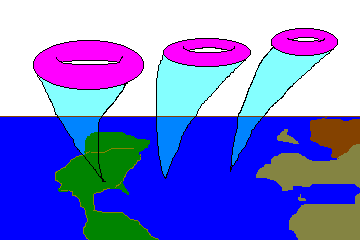This is actually a very old idea dating back to the 1920's and the work of Kaluza and Klein. This mechanism is often called Kaluza-Klein theory or compactification. In the original work of Kaluza it was shown that if we start with a theory of general relativity in 5-spacetime dimensions and then curl up one of the dimensions into a circle we end up with a 4-dimensional theory of general relativity plus electromagnetism! The reason why this works is that electromagnetism is a U(1) gauge theory, and U(1) is just the group of rotations around a circle. If we assume that the electron has a degree of freedom corresponding to point on a circle, and that this point is free to vary on the circle as we move around in spacetime, we find that the theory must contain the photon and that the electron obeys the equations of motion of electromagnetism (namely Maxwell's equations). The Kaluza-Klein mechanism simply gives a geometrical explanation for this circle: it comes from an actual fifth dimension that has been curled up. In this simple example we see that even though the compact dimensions maybe too small to detect directly, they still can have profound physical implications. [Incidentally the work of Kaluza and Klein leaked over into the popular culture launching all kinds of fantasies about the "Fifth dimension"!]

How would we ever really know if there were extra dimensions and how could we detect them if we had particle accelerators with high enough energies? From quantum mechanics we know that if a spatial dimension is periodic the momentum in that dimension is quantized, p = n / R (n=0,1,2,3,....), whereas if a spatial dimension is unconstrained the momentum can take on a continuum of values. As the radius of the compact dimension decreases (the circle becomes very small) then the gap between the allowed momentum values becomes very wide. Thus we have a Kaluza Klein tower of momentum states.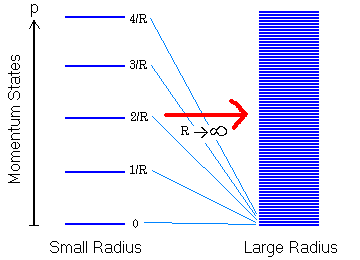If we take the radius of the circle to be very large (the dimension is de-compactifying) then the allowed values of the momentum become very closely spaced and begin to form a continuum. These Kaluza-Klein momentum states will show up in the mass spectrum of the uncompactifed world. In particular, a massless state in the higher dimensional theory will show up in the lower dimensional theory as a tower of equally spaced massive states just as in the picture shown above. A particle accelerator would then observe a set of particles with masses equally spaced from each other. Unfortunately, we'd need a very high energy accelerator to see even the lightest massive particle.

Strings have a fascinating extra property when compactified: they can wind around a compact dimension which leads to winding modes in the mass spectrum. A closed string can wind around a periodic dimension an integral number of times. Similar to the Kaluza-Klein case they contribute a momentum which goes as p = w R (w=0,1,2,...). The crucial difference here is that this goes the other way with respect to the radius of the compact dimension, R. So now as the compact dimension becomes very small these winding modes are becoming very light!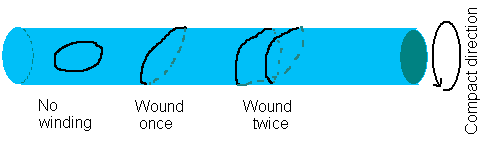Now to make contact with our 4-dimensional world we need to compactify the 10-dimensional superstring theory on a 6-dimensional compact manifold. Needless to say, the Kaluza Klein picture described above becomes a bit more complicated. One way could simply be to put the extra 6 dimensions on 6 circles, which is just a 6-dimensional Torus. As it turns out this would preserve too much supersymmetry. It is believed that some supersymmetry exists in our 4-dimensional world at an energy scale above 1 TeV (this is the focus of much of the current and future research at the highest energy accelerators around the word!). To preserve the minimal amount of supersymmetry, N=1 in 4 dimensions, we need to compactify on a special kind of 6-manifold called a Calabi-Yau manifold.

The properties of the Calabi-Yau manifold can have important implications for low energy physics such as the types of particles observed, their masses and quantum numbers, and the number of generations.  One of the outstanding problems in the field has been the fact that there are many many Calabi-Yau manifolds (thousands upon thousands?) and we have no way of knowing which one to use.  In a sense we started with a virtually unique 10-dimensional string theory and have found that possibilities for 4-dimensional physics are far from unique, at least at the level of our current (and incomplete) understanding. The long-standing hope of string theorists is that a detailed knowledge of the full non-perturbative structure of the theory, will lead us to an explanation of how and why our universe flowed from the 10-dimensional physics that probably existed during the high energy phase of the Big Bang, down to the low energy 4-dimensional physics that we observe today. [Possibly we will find a unique Calabi-Yau manifold that does the trick.] Some important work of Andrew Strominger has shown that Calabi-Yau manifolds can be continuously connected to one another through conifold transitions and that we can move between different Calabi-Yau manifolds by varying parameters in the theory.  This suggests the possibility that the various 4-dimensional theories arising from different Calabi-Yau manifolds might actually be different phases of an single underlying theory.

PreviousNextContents

| Contact and Feedback |